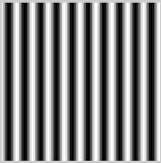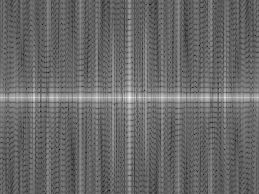## Friday, June 26, 2015

### 2D FFT: Binarization of 2D FFT Spectra of Image Waves of Different Frequencies and Orientations

Problem

This is a list of posts on the binarization of the 2D FFT frequency spectra of wave images of different frequencies and orientations. I could not put all images into a single post and had to break it into four different posts each of which contains an example of how to binarize the 2D FFT of wave images.

List of Blog Posts

### 2D FFT: Binarization of 2D FFT of Wave Images: Part 04

Problem

This post continues our series of posts on a programmatic investigation of the 2D FFT. In this post, we will address the question of computing 2D FFT of wave images and binarizing them to obtain the main pattern. The slide presentation for this post is available here.The previous post on the 2D FFT of wave images is here.

Wave Images of Different Frequencies

Figure 1 shows an wave image.Figure 1. 2D_wave_04.png
Our 2D FFT analysis of images, such as the one shown in Figure 1, is done with the following Matlab code.

figure;
imshow(wave_04);
title('Wave 04');

%% Do 2D FFT
wave_04_fft = fft2(double(wave_04)); %% convert image into double and apply 2D FFT to it
wave_04_fft = fftshift(wave_04_fft); %% shift the frequency spectrum's origin into the middle

wave_04_fft = abs(wave_04_fft); %% compute the magnitudes of the obtained transformed
wave_04_fft = log(wave_04_fft+1); %% transform the 2D FFT into the logspace
wave_04_fft = mat2gray(wave_04_fft); %% grayscale

figure;
imshow(wave_04_fft,[]); %% Display the result shown in Figure 2 below.
title('Wave 04 2D FFT Magn');

%% Find the maximum value and its location in the spectrum
[value_max, location_max] = max(wave_04_fft(:));
[row_max, col_max] = ind2sub(size(wave_04_fft), location_max);
%% Find the maximum value and its location in the spectrum
[value_min, location_min] = min(wave_04_fft(:));
[row_min, col_min] = ind2sub(size(wave_04_fft), location_min);
%% Threshold and binarize
wave_04_fft_max = (wave_04_fft >= 0.90);
wave_04_fft_min = (wave_04_fft_max <= 0.1);
wave_04_fft(wave_04_fft_max) = 255;
wave_04_fft(wave_04_fft_min) = 0;
%% Shown in Figure 3 below.
imshow(wave_04_fft, []);
title('Wave 04 2D FFT Threshed');

Figure 2 displays the unthresholded result of the 2D FFT.Figure 2. Unthresholded 2D FFT of the image in Figure 1

Figure 3 below shows the thresholded and binarized version of the 2D FFT spectrum shown in Figure 2.  The center dot shows the complex 2D sinusoid with 0 frequencies along the x and y axes. The right dot corresponds to a complex sinusoid whose magnitude corresponds to the frequency of the wave in Figure 1 moving east. The bottom right point corresponds to another complex sinusoid whose magnitude corresponds to the frequency of the wave in Figure 1 moving
west.Figure 3. Thresholed and binarized 2D FFT of the image in Figure 1